#115 Doe Purebred

BW = 4.6lbs

30 Day = 12.2 = .26adg

60 Day = 23.8 = .32adg

90 Day = 31.0 = .29adg

3/6/21 Kentucky's Bourbon triplets. Two girls and a boy.

Sire: DOG Hamish

Kids can be registered through NKR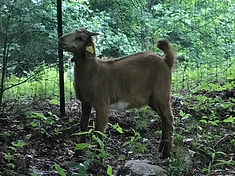#116 Wether Purebred

BW = 7.2lbs

30 Day = 19.2 = .40adg

60 Day = 32.8 = .43adg

90 Day = 36.8 = .33adg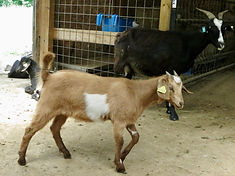#117 Doe Purebred

BW = 5.0lbs

30 Day = 14.2 = .31adg

60 Day = 25.8 = .35adg

90 Day = 41.4 = .40adg3/8/21 Odin's Powder Blue again twinned girls.

Sire: RHK Strike's War Paint

Kids can be registered through NKR or AKGA#118 Doe 100%New Zealand

BW = 6.2lbs

30 Day = 19.4 = .44adg

60 Day = 29.4 = .39adg

90 Day = 41.4 = .39adg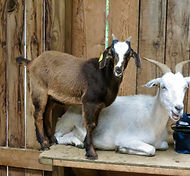#119 Doe 100% New Zealand

BW = 6.4lbs

30 Day = 19.4 = .43adg

60 Day = 30.4 = .40adg

90 Day = 40.4 = .38adg#120 Buck Purebred

BW = 8.6lbs

30 Day = 22.4 = .46adg

60 Day = 38.0 = .49adg

90 Day = 55.0 = .52adg

3/8321 HMK Jocelyn kidded triplets, unfortunately one was still born. Here she is pictured with her buck and doe.

Sire: RHK Strike's War Paint

Kids can be registered through NKR#121 Doe Purebred

BW = 6.8lbs

30 Day = 19.6 = .43adg

60 Day = 31.6 = .41adg

90 Day = 42.0 = .39adg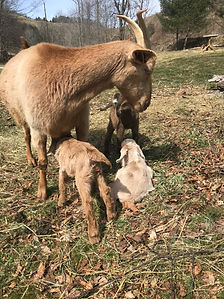3/9/21 SMK Jasmine kidded triplets 2 does & 1 buck

Sire: DOG Hamish

Kids can be registered through NKR#122 Doe 100% New Zealand

BW = 6.6lbs

30 Day = 20.8 = .47adg

60 Day = 32.6 = .43adg

90 Day = 43.6 = .41adg

#123 Buck 100% New Zealand

BW = 6.4lbs

30 Day = 22.0 = .52adg

60 Day = 40.2 = .56adg

90 Day = 58.0 = .57adg

#124 Doe 100% New Zealand

BW = 5.6lbs

30 Day = 18.2 = .42adg

60 Day = 33.2 = .46adg

90 Day = 46.0 = .45adg#125 Doe Purebred (white)

BW = 7.4lbs

30 Day = 20.8 = .45adg

60 Day = 35.2 = .46adg

90 Day = 46.8 = .44adg

3/9/21 DOG Tove kidded twin doelings

Sire: DOG Hamish

Kids can be registered through NKR#126 Doe Purebred (brown)

BW = 7.0lbs

30 Day = 19.2 = .41adg

60 Day = 32.2 = .42adg

90 Day = 42.8 = .40adg#127 Doe 100% New Zealand

BW = 5.2lbs

30 Day = 14.8 = .32adg

60 Day = 24.4 = .32adg

90 Day = 33.8 = .32adg

3/9/21 RDH Isaiah's Roho Vision kidded triplet girls.

Sire: RHK Strike's War Paint

Kids can be registered through

NKR or AKGA#128 Doe 100% New Zealand

BW = 6.8lbs

30 Day = 18.2 = .38adg

60 Day = 27.6 = .35adg

90 Day = 38.4 = .35adg#129 Doe 100% New Zealand

BW = 6.6lbs

30 Day = 16.8 = .34adg

60 Day = 27.0 = .34adg

90 Day = 36.0 = .33adg#130 Buck 100% New Zealand

BW = 9.2lbs

30 Day = 26 = .56adg

60 Day = 42.2 = .55adg

90 Day = 55.5 = .52adg

3/10/21 DRG Onyx's Wicked Powder kidded twins, a buck and a doe

Sire: DOG Hamish

Kids can be registered through NKR#131 Doe 100% New Zealand

BW = 8.4lbs

30 Day = 23.2 = .49adg

60 Day = 39.2 = .51adg

90 Day = 55.4 = .52adg#132 Doe Purebred

BW = 9.0lbs

30 Day = 25 = .53adg

60 Day = 41 = .53adg

90 Day = 54 = .50adg

3/11/21 CBK Freyja kidded a doe

Sire: RHK Strike's War Paint

Doe can be registered through NKR3/12/21 RDH Isaiah's Black Satin kidded triplet bucks.

Sire: RHK Strike's War Paint

Kids can be registered through NKR or AKGA#133 Buck 100% New Zealand

BW = 8.0lbs

30 Day = 21.8 = .46adg

60 Day = 38.2 = .50adg

90 Day = 51.4 = .48adg#134 Buck 100% New Zealand

BW = 7.8lbs

30 Day = 21.8 = .47adg

60 Day = 37.2 = .49adg

90 Day = 50.4 = .47adg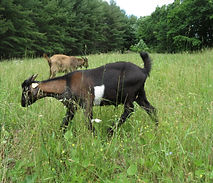#135 Buck 100% New Zealand

BW = 7.8lbs

30 Day = 21.2 = .45adg

60 Day = 35.6 = .46adg

90 Day = 49.6 = .46adg#136 Buck Purebred

BW = 8.0lbs

30 Day = 22.2 = .47adg

60 Day = 37.0 = .47adg

90 Day = 49.4 = .46adg

3/16/21 WHF Lucie kidded triplet bucks

Sire: RHK Strike's War Paint

Kids can be registered NKR or AKGA#137 Buck Purebred

BW = 7.2lbs

30 Day = 18.6 = .38adg

60 Day = 32.2 = .40adg

90 Day = 45.8 = .43adg#138 Buck Purebred

BW = 7.8lbs

30 Day = 20.2 = .41adg

60 Day = 39.0 = .50adg

90 Day = 53.0 = .50adg#139 Buck 100% New Zealand

BW = 11.8lbs

30 Day = 26 = .46adg

60 Day = 44 = .53adg

90 Day = 62.2 = .56adg

3/17/21 LFK Outlaw's Gloria kidded twins, a buck and doe.

Sire: RHK Strike's War Paint

kids can be registered NKR or AKGA

#140 Doe 100% New Zealand

BW 8.6lbs

Unfortunately we lost her#141 Buck Purebred

BW = 9lbs

30 Day = 26.4 = .58adg

60 Day = 44.6 = .59adg

90 Day = 60.2 = .57adg

3/27/21HMK Jolie kidded triplets,  unfortunately, one was still born, but pictured here with

her buck and doe

Sire: RHK Strike's War Paint

kids can be registered NKR#142 Doe Purebred

BW = 7.6lbs

30 Day = 22.2 = .49adg

60 Day = 38.0 = .51adg

90 Day = 47.0 = .44adg